Nuclear Physics

# College Physics Reasoning and Relationships

Physics & Astronomy

## Quiz 30 :Nuclear PhysicsStudy FlashcardsLooking for Introductory Physics Homework Help?## Quiz 30 :Nuclear Physics

Showing 1 - 20 of 70The nucleus of an atom is made up of which of the following?
Free
Multiple Choice

BThe experiment, which gave the first evidence for the existence of the atomic nucleus, involved which of the following?
Free
Multiple Choice

DThe atomic number of a given element is equivalent to which of the following?
Free
Multiple Choice

CRutherford's experiments involving the use of alpha particle beams directed onto thin metal foils demonstrated the existence of which of the following?
Multiple ChoiceThe atomic mass number of a nucleus is equivalent to which of the following numbers?
Multiple ChoiceThe isotope 64Zn has a nuclear radius of 4.8 1015 m. Approximately what is the nuclear radius of the isotope 125Te?
Multiple ChoiceThe isotope 64Zn has a nuclear radius of 4.8 1015 m. Which of the following is the mass number of an isotope for which the nuclear radius is 6.8 1015 m?
Multiple ChoiceIf there are 146 neutrons in 238U, how many neutrons are found in the nucleus of 233U?
Multiple ChoiceThe mass of 12C is 12 u, where 1 u = 1.660559 1027 kg. This mass is equal to:
Multiple ChoiceFor every stable nucleus except hydrogen, if the number of nucleons is doubled, the quantity that will change least is the:
Multiple ChoiceThe molecular mass for chlorine is not an integer multiple of the molecular mass of hydrogen but is about 35.5 times the molecular mass of hydrogen. This is primarily because:
Multiple ChoiceCertain stars at the end of their lives are thought to collapse, combining their protons and electrons together to form a neutron star. Such a star could be thought of as a giant atomic nucleus. If a star of mass equal to M = 4.00 1030 kg collapsed into neutrons (mn = 1.67 1027 kg), what would be the radius of such a star? (Hint: r = r0A1/3, where r0 = 1.20 1015 m.)
Multiple ChoiceThe relationship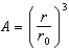, where A is the mass number of a nucleus and r is it radius, implies which of the following about nuclei?
Multiple ChoiceWhat potential difference is required to accelerate the nucleus of the atom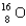, initially at rest, to a kinetic energy of 4.0 MeV?
Multiple ChoiceSample #1 is made from an isotope with decay constant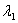and sample #2 is made from an isotope with decay constant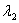, where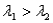. Which of the following statements must be true?
Multiple ChoiceTwo different nuclei emit alpha particles, the energy released in each of these decays being the same. Which of the following has the lowest resulting kinetic energy?
Multiple ChoiceAlpha radiation is in fact which of the following?
Multiple ChoiceGamma radiation is in fact which of the following?
Multiple Choice